#### Tests and Measures of Agreement

When you specify the AGREE option in the TABLES statement, PROC FREQ computes tests and measures of agreement for square tables (that is, for tables where the number of rows equals the number of columns). For two-way tables, these tests and measures include McNemar’s test fortables, Bowker’s test of symmetry, the simple kappa coefficient, and the weighted kappa coefficient. For multiple strata (n-way tables, where n > 2), PROC FREQ also computes the overall simple kappa coefficient and the overall weighted kappa coefficient, as well as tests for equal kappas (simple and weighted) among strata. Cochran’s Q is computed for multiway tables when each variable has two levels, that is, for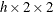tables.

PROC FREQ computes the kappa coefficients (simple and weighted), their asymptotic standard errors, and their confidence limits when you specify the AGREE option in the TABLES statement. If you also specify the KAPPA option in the TEST statement, then PROC FREQ computes the asymptotic test of the hypothesis that simple kappa equals zero. Similarly, if you specify the WTKAP option in the TEST statement, PROC FREQ computes the asymptotic test for weighted kappa.

In addition to the asymptotic tests described in this section, PROC FREQ provides exact p-values for McNemar’s test, the simple kappa coefficient test, and the weighted kappa coefficient test. You can request these exact tests by specifying the corresponding options in the EXACT statement. See the section Exact Statistics for more information.

The following sections provide the formulas that PROC FREQ uses to compute the AGREE statistics. For information about the use and interpretation of these statistics, see Agresti (2002, 2007); Fleiss, Levin, and Paik (2003), and the other references cited for each statistic.

##### McNemar’s Test

PROC FREQ computes McNemar’s test fortables when you specify the AGREE option. McNemar’s test is appropriate when you are analyzing data from matched pairs of subjects with a dichotomous (yes-no) response. It tests the null hypothesis of marginal homogeneity, or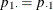. McNemar’s test is computed as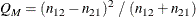Under the null hypothesis,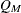has an asymptotic chi-square distribution with one degree of freedom. See McNemar (1947), as well as the general references cited in the preceding section. In addition to the asymptotic test, PROC FREQ also computes the exact p-value for McNemar’s test when you specify the MCNEM option in the EXACT statement.

##### Bowker’s Test of Symmetry

For Bowker’s test of symmetry, the null hypothesis is that the cell proportions are symmetric, or that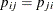for all pairs of table cells. Fortables, Bowker’s test is identical to McNemar’s test, and so PROC FREQ provides Bowker’s test for square tables larger than.

Bowker’s test of symmetry is computed as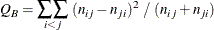For large samples,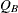has an asymptotic chi-square distribution with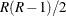degrees of freedom under the null hypothesis of symmetry. See Bowker (1948) for details.

##### Simple Kappa Coefficient

The simple kappa coefficient, introduced by Cohen (1960), is a measure of interrater agreement. PROC FREQ computes the simple kappa coefficient as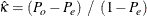where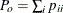and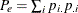. If the two response variables are viewed as two independent ratings of the n subjects, the kappa coefficient equals +1 when there is complete agreement of the raters. When the observed agreement exceeds chance agreement, kappa is positive, with its magnitude reflecting the strength of agreement. Although this is unusual in practice, kappa is negative when the observed agreement is less than chance agreement. The minimum value of kappa is between –1 and 0, depending on the marginal proportions.

The asymptotic variance of the simple kappa coefficient is computed as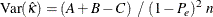where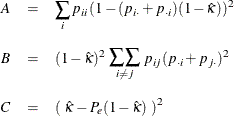See Fleiss, Cohen, and Everitt (1969) for details.

PROC FREQ computes confidence limits for the simple kappa coefficient as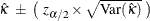where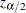is the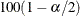percentile of the standard normal distribution. The value ofis determined by the value of the ALPHA= option, which, by default, equals 0.05 and produces 95% confidence limits.

To compute an asymptotic test for the kappa coefficient, PROC FREQ uses the standardized test statistic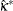, which has an asymptotic standard normal distribution under the null hypothesis that kappa equals zero. The standardized test statistic is computed as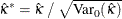where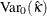is the variance of the kappa coefficient under the null hypothesis,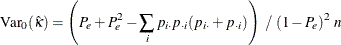See Fleiss, Levin, and Paik (2003) for details.

PROC FREQ also provides an exact test for the simple kappa coefficient. You can request the exact test by specifying the KAPPA or AGREE option in the EXACT statement. See the section Exact Statistics for more information.

##### Weighted Kappa Coefficient

The weighted kappa coefficient is a generalization of the simple kappa coefficient that uses weights to quantify the relative difference between categories. Fortables, the weighted kappa coefficient equals the simple kappa coefficient. PROC FREQ displays the weighted kappa coefficient only for tables larger than. PROC FREQ computes the kappa weights from the column scores, by using either Cicchetti-Allison weights or Fleiss-Cohen weights, both of which are described in the following section. The weights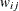are constructed so that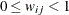for all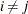,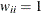for all i, and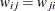. The weighted kappa coefficient is computed as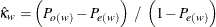where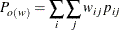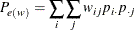The asymptotic variance of the weighted kappa coefficient is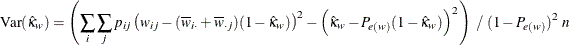where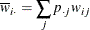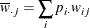See Fleiss, Cohen, and Everitt (1969) for details.

PROC FREQ computes confidence limits for the weighted kappa coefficient as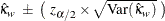whereis thepercentile of the standard normal distribution. The value ofis determined by the value of the ALPHA= option, which, by default, equals 0.05 and produces 95% confidence limits.

To compute an asymptotic test for the weighted kappa coefficient, PROC FREQ uses the standardized test statistic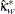, which has an asymptotic standard normal distribution under the null hypothesis that weighted kappa equals zero. The standardized test statistic is computed as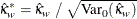where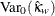is the variance of the weighted kappa coefficient under the null hypothesis,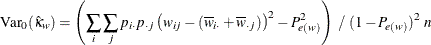See Fleiss, Levin, and Paik (2003) for details.

PROC FREQ also provides an exact test for the weighted kappa coefficient. You can request the exact test by specifying the WTKAPPA or AGREE option in the EXACT statement. See the section Exact Statistics for more information.

###### Weights

PROC FREQ computes kappa coefficient weights by using the column scores and one of the two available weight types. The column scores are determined by the SCORES= option in the TABLES statement. The two available types of kappa weights are Cicchetti-Allison and Fleiss-Cohen weights. By default, PROC FREQ uses Cicchetti-Allison weights. If you specify (WT=FC) with the AGREE option, then PROC FREQ uses Fleiss-Cohen weights to compute the weighted kappa coefficient.

PROC FREQ computes Cicchetti-Allison kappa coefficient weights as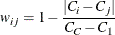where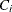is the score for column i and C is the number of categories or columns. See Cicchetti and Allison (1971) for details.

The SCORES= option in the TABLES statement determines the type of column scores used to compute the kappa weights (and other score-based statistics). The default is SCORES=TABLE. See the section Scores for details. For numeric variables, table scores are the values of the variable levels. You can assign numeric values to the levels in a way that reflects their level of similarity. For example, suppose you have four levels and order them according to similarity. If you assign them values of 0, 2, 4, and 10, the Cicchetti-Allison kappa weights take the following values: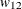= 0.8,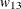= 0.6,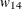= 0,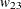= 0.8,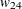= 0.2, and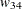= 0.4. Note that when there are only two categories (that is, C = 2), the weighted kappa coefficient is identical to the simple kappa coefficient.

If you specify (WT=FC) with the AGREE option in the TABLES statement, PROC FREQ computes Fleiss-Cohen kappa coefficient weights as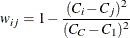See Fleiss and Cohen (1973) for details.

For the preceding example, the Fleiss-Cohen kappa weights are:= 0.96,= 0.84,= 0,= 0.96,= 0.36, and= 0.64.

##### Overall Kappa Coefficient

When there are multiple strata, PROC FREQ combines the stratum-level estimates of kappa into an overall estimate of the supposed common value of kappa. Assume there are q strata, indexed by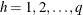, and let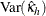denote the variance of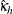. The estimate of the overall kappa coefficient is computed as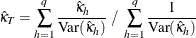See Fleiss, Levin, and Paik (2003) for details.

PROC FREQ computes an estimate of the overall weighted kappa in the same way.

##### Tests for Equal Kappa Coefficients

When there are multiple strata, the following chi-square statistic tests whether the stratum-level values of kappa are equal: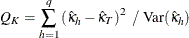Under the null hypothesis of equal kappas for the q strata,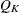has an asymptotic chi-square distribution with q–1 degrees of freedom. See Fleiss, Levin, and Paik (2003) for more information. PROC FREQ computes a test for equal weighted kappa coefficients in the same way.

##### Cochran’s Q Test

Cochran’s Q is computed for multiway tables when each variable has two levels, that is, for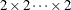tables. Cochran’s Q statistic is used to test the homogeneity of the one-dimensional margins. Let m denote the number of variables and N denote the total number of subjects. Cochran’s Q statistic is computed as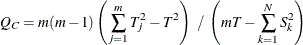where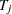is the number of positive responses for variable j, T is the total number of positive responses over all variables, and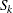is the number of positive responses for subject k. Under the null hypothesis, Cochran’s Q has an asymptotic chi-square distribution with m–1 degrees of freedom. See Cochran (1950) for details. When there are only two binary response variables (m=2), Cochran’s Q simplifies to McNemar’s test. When there are more than two response categories, you can test for marginal homogeneity by using the repeated measures capabilities of the CATMOD procedure.

##### Tables with Zero Rows and Columns

The AGREE statistics are defined only for square tables, where the number of rows equals the number of columns. If the table is not square, PROC FREQ does not compute AGREE statistics. In the kappa statistic framework, where two independent raters assign ratings to each of n subjects, suppose one of the raters does not use all possible r rating levels. If the corresponding table has r rows but only r–1 columns, then the table is not square and PROC FREQ does not compute AGREE statistics. To create a square table in this situation, use the ZEROS option in the WEIGHT statement, which requests that PROC FREQ include observations with zero weights in the analysis. Include zero-weight observations in the input data set to represent any rating levels that are not used by a rater, so that the input data set has at least one observation for each possible rater and rating combination. The analysis then includes all rating levels, even when all levels are not actually assigned by both raters. The resulting table (of rater 1 by rater 2) is a square table, and AGREE statistics can be computed.

For more information, see the description of the ZEROS option. By default, PROC FREQ does not process observations that have zero weights, because these observations do not contribute to the total frequency count, and because any resulting zero-weight row or column causes many of the tests and measures of association to be undefined. However, kappa statistics are defined for tables with a zero-weight row or column, and the ZEROS option makes it possible to input zero-weight observations and construct the tables needed to compute kappas.